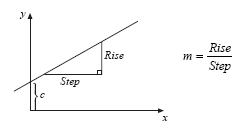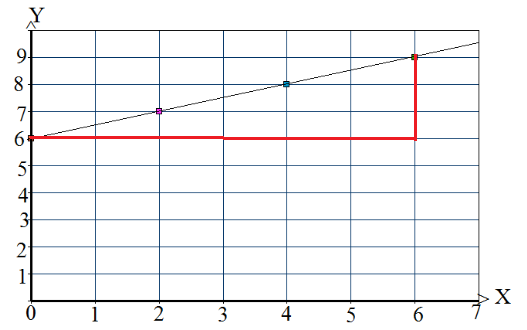# Line of Best Fit Formula

A line of best fit is a straight line that is the best approximation of the given set of data. In simple term, it is a graphical representation

A more accurate way of finding the line of best fit is the least square method. If you draw a line of best fit, it is possible to determine the equation of the line of best fit. You will remember that the equation of a straight line is given by

$\large y=mx+c$

Where,
c is the intercept

##Solved Example

Example: The points with coordinates (0, 6), (2, 7), (4, 8) and (6, 9) lie on a straight line. Draw the line and determine its equation.

Solution

The following graph shows a scatter plot and a line of best fit:m=3/6 = 1/2

Equation: y = (1/2)x +6